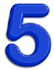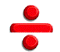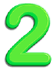# Is teaching “mixed numbers” a stupid idea?I thought about this quote from Paul Lockhart for a long time today, trying to decide whether I agreed with it or not.

“No mathematician in the world would bother making these senseless distinctions: 2 1/2 is a “mixed number ” while 5/2 is an “improper fraction.” They’re EQUAL for crying out loud. They are the exact same numbers and have the exact same properties. Who uses such words outside of fourth grade?”

I wasn’t thinking about whether 2 1/2 and 5/2 are the same numbers and have the same properties, because that part is self-evident and would be silly to argue. Is there a point in teaching children terms and marking them wrong if they put the answer as a mixed number instead a fraction?

I’m inclined to agree with Lockhart in one way because there are a lot of things we do in teaching statistics that seem stupid to me. For example, statistics textbooks often have loads of homework problems where students are required to use a calculator to compute sums of squares for Analysis of Variance. You ask any working statistician when is the last time he or she did that and you will get laughter in your face because it is 2015 and we are grown-ups and we use computers.

What is important to know is that total variance can be partitioned into explained variance and unexplained variance. It is further important to know that explained variance in a model can be broken down into the amount of variance explained by each term.This is where I wonder, though, if it is possible that I am wrong and Lockhart is wrong and other people are right. When I was in graduate school, I actually computed sums of squares, z-scores etc. with a pencil and a piece of paper because I am that old and I was that broke back then. When I finally got a calculator about the third year into my Ph.D. program – well, it’s been nearly 30 years and I still remember how happy I was.

Maybe having to get down into that level of detail made me understand better that a correlation of 1.0 does not mean that the scores on two variables are identical but rather that their z-scores are identical.

I will admit in Fish Lake that there are a couple of places that students are specifically asked to write , say,the product of

5 x 1/2

as a mixed number and then write it again as an improper fraction. The whole point of those exercises are to emphasize exactly what Lockhart says, which is that the two ARE the exact same thing. How are you going to know that unless someone teaches you? At some point we have probably forgotten, we all learned that

2.5 = 5/2 =  2 1/2

There are different ways of writing numbers that all mean the same thing. Two wholes plus one-half is the same as five halves.

It seems to me at least possible that the way you think about something after you have learned it so deeply that you just take it for granted is perhaps not the same way you talk and think about that thing when you are just learning it for the first time.